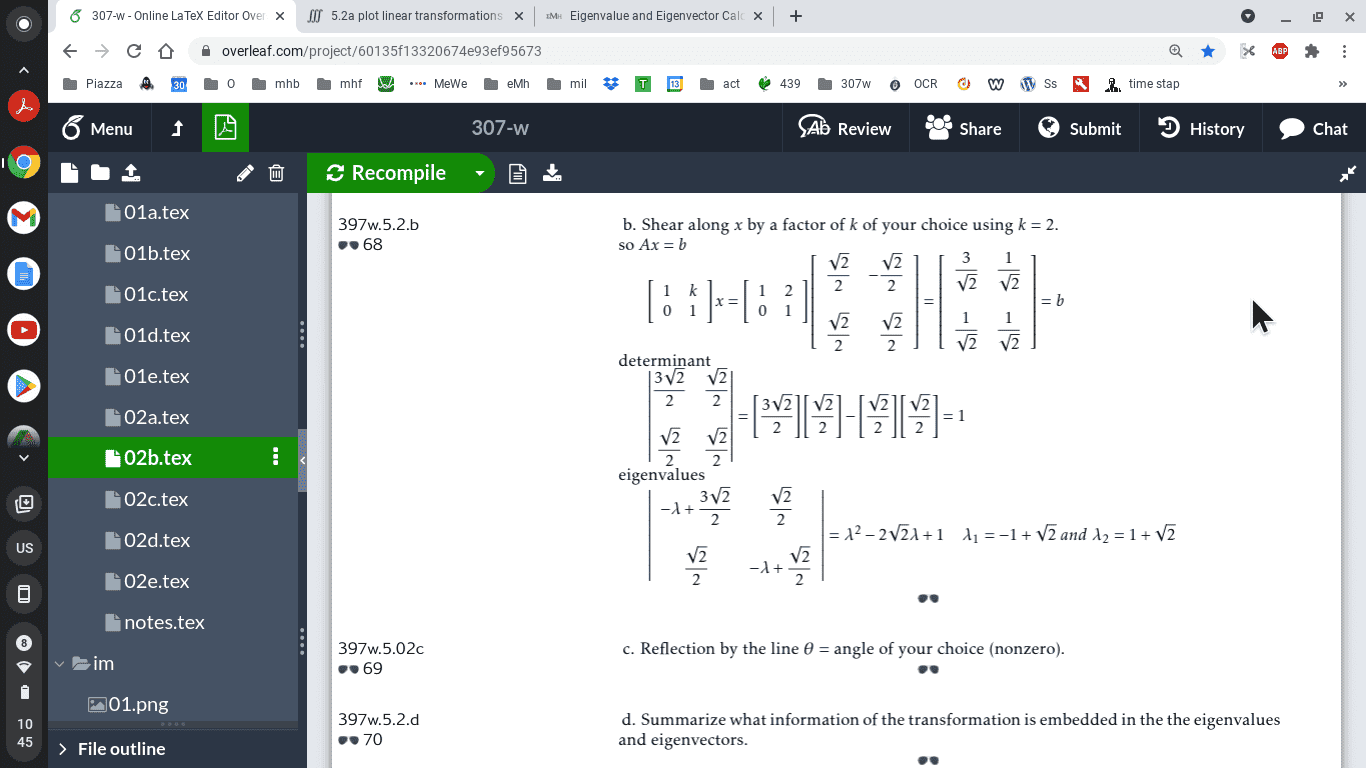# 5.2a plot linear transformations

• MHB
Gold Member
MHB
ok we are supposed to go to here

Find 3 different matrices that reflect the following transformations, report the matrix, the determinant, and the eigenvalues.

1. Rotation by $\dfrac{\pi}{4}$
2. Shear along $x$ by a factor of $k$
3. Reflection by the line $\theta$

there are some more but the site asked for doesn't return the transformed matrix just a morphed image
also I presume we doing all 3 matrices with just the matrix's used in the site

I tried geogebra and W|A also but the plots didn't look like vectors

anyway this is due on Friday so hope I can get it right. I am sure the answer is relatively simple
,

Last edited:

probab'y would have been better for $k=\sqrt{2}$